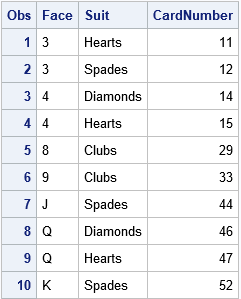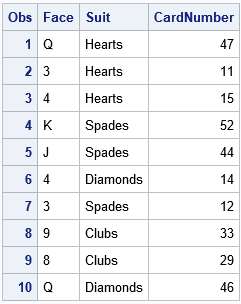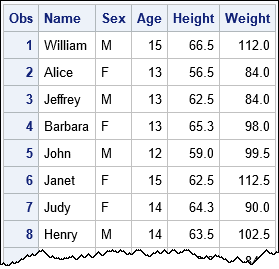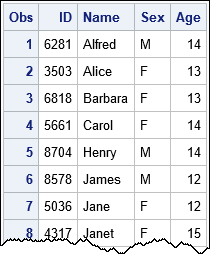The SURVEYSELECT procedure in SAS 9.4M5 supports the OUTRANDOM option, which causes the selected items in a simple random sample to be randomly permuted after they are selected. This article describes several statistical tasks that benefit from this option, including simulating card games, randomly permuting observations in a DATA step, assigning a random ID to patients in a clinical study, and generating bootstrap samples. In each case, the new OUTRANDOM option reduces the number of statements that you need to write. The OUTRANDOM option can also be specified by using OUTORDER=RANDOM.

### Sample data with PROC SURVEYSELECT

Often when you draw a random sample (with or without replacement) from a population, the order in which the items were selected is not important. For example, if you have 10 patients in a clinical trial and want to randomly assign five patients to the control group, the control group does not depend on the order in which the patients were selected. Similarly, in simulation studies, many statistics (means, proportions, standard deviations,...) depend only on the sample, not on the order in which the sample was generated. For these reasons, and for efficiency, the SURVEYSELECT procedure in SAS uses a "one-pass" algorithm to select observations in the same order that they appear in the "population" data set.

However, sometimes you might require the output data set from PROC SURVEYSELECT to be in a random order. For example, in a poker simulation, you might want the output of PROC SURVEYSELECT to represent a random shuffling of the 52 cards in a deck. To be specific, the following DATA step generates a deck of 52 cards in order: Aces first, then 2s, and so on up to jacks, queens, and kings. If you use PROC SURVEYSELECT and METHOD=SRS to select 10 cards at random (without replacement), you obtain the following subset:

```data CardDeck; length Face \$2 Suit \$8; do Face = 'A','2','3','4','5','6','7','8','9','10','J','Q','K'; do Suit = 'Clubs', 'Diamonds', 'Hearts', 'Spades'; CardNumber + 1; output; end; end; run;   /* Deal 10 cards. Order is determined by input data */ proc surveyselect data=CardDeck out=Deal noprint seed=1234 method=SRS /* sample w/o replacement */ sampsize=10; /* number of observations in sample */ run;   proc print data=Deal; run;```Notice that the call to PROC SURVEYSELECT did not use the OUTRANDOM option. Consequently, the cards are in the same order as they appear in the input data set. This sample is adequate if you want to simulate dealing hands and estimate probabilities of pairs, straights, flushes, and so on. However, if your simulation requires the cards to be in a random order (for example, you want the first five observations to represent the first player's cards), then clearly this sample is inadequate and needs an additional random permutation of the observations. That is exactly what the OUTRANDOM option provides, as shown by the following call to PROC SURVEYSELECT:

```/* Deal 10 cards in random order */ proc surveyselect data=CardDeck out=Deal2 noprint seed=1234 method=SRS /* sample w/o replacement */ sampsize=10 /* number of observations in sample */ OUTRANDOM; /* SAS/STAT 14.3: permute order */ run;   proc print data=Deal2; run;```You can use this sample when the output needs to be in a random order. For example, in a poker simulation, you can now assign the first five cards to the first player and the second five cards to a second player.

### Permute the observations in a data set

A second application of the OUTRANDOM option is to permute the rows of a SAS data set. If you sample without replacement and request all observations (SAMPRATE=1), you obtain a copy of the original data in random order. For example, the students in the Sashelp.Class data set are listed in alphabetical order by their name. The following statements use the OUTRANDOM option to rearrange the students in a random order:

```/* randomly permute order of observations */ proc surveyselect data=Sashelp.Class out=RandOrder noprint seed=123 method=SRS /* sample w/o replacement */ samprate=1 /* proportion of observations in sample */ OUTRANDOM; /* SAS/STAT 14.3: permute order */ run;   proc print data=RandOrder; run;```There are many other ways to permute the rows of a data set, such as adding a uniform random variable to the data and then sorting. The two methods are equivalent, but the code for the SURVEYSELECT procedure is shorter to write.

### Assign unique random IDs to patients in a clinical trial

Another application of the OUTRANDOM option is to assign a unique random ID to participants in an experimental trial. For example, suppose that four-digit integers are used for an ID variable. Some clinical trials assign an ID number sequentially to each patient in the study, but I recently learned from a SAS discussion forum that some companies assign random ID values to subjects. One way to assign random IDs is to sample randomly without replacement from the set of all ID values. The following DATA step generates all four-digit IDs, selects 19 of them in random order, and then merges those IDs with the participants in the study:

```data AllIDs; do ID = 1000 to 9999; /* create set of four-digit ID values */ output; end; run;   /* randomly select 19 unique IDs */ proc surveyselect data=AllIDs out=ClassIDs noprint seed=12345 method=SRS /* sample w/o replacement */ sampsize=19 /* number of observations in sample */ OUTRANDOM; /* SAS/STAT 14.3: permute order */ run;   data Class; merge ClassIDs Sashelp.Class; /* merge ID variable and subjects */ run;   proc print data=Class; var ID Name Sex Age; run;```### Random order for other sampling methods

The OUTRANDOM option also works for other sampling schemes, such as sampling with replacement (METHOD=URS, commonly used for bootstrap sampling) or stratified sampling. If you use the REPS= option to generate multiple samples, each sample is randomly ordered.

It is worth mentioning that the SAMPLE function in SAS/IML also can to perform a post-selection sort. Suppose that X is any vector that contains N elements. Then the syntax SAMPLE(X, k, "NoReplace") generates a random sample of k elements from the set of N. The documentation states that "the elements ... might appear in the same order as in X." This is likely to happen when k is almost equal to N. If you need the sample in random order, you can use the syntax SAMPLE(X, k, "WOR") which adds a random sort after the sample is selected, just like PROC SURVEYSELECT does when you use the OUTRANDOM option.

The post Sample and obtain the results in random order appeared first on The DO Loop.